# Simple yet interesting.

## Recommended Posts

Just for you Ghideon I simplified the equation to prove you do understand it. It has been said if you can’t explain it simply you don’t understand it.

Remember there is no guarantee it works, but it is simple enough to test.

x^3 = [(x^2 * pnp^2) / (pnp^2 + x)]

try pnp=85 and an x of 5

Then test more values.

Again it may not work but you have followed my thread and challenged me to simplify and prove my hypothesis.

• Replies 95
• Created

#### Posted Images

5 hours ago, Trurl said:

Remember there is no guarantee it works, but it is simple enough to test.

x^3 = [(x^2 * pnp^2) / (pnp^2 + x)]

try pnp=85 and an x of 5

Then test more values.

What is the definition of the test, a fail and a pass?

Note: The expression $x^{3} = \frac {x^2 * pnp^{2}}{ pnp^{2} + x}$ does not hold for pnp=85 and and x=5.

##### Share on other sites

Sorry try

Remember there is no guarantee it works, but it is simple enough to test.

x^3 = [(x^3 * pnp^2) / (pnp^2 + x)]

try pnp=85 and an x of 5

Then test more values.

##### Share on other sites

Posted (edited)
20 hours ago, Trurl said:

Sorry try

Remember there is no guarantee it works, but it is simple enough to test.

x^3 = [(x^3 * pnp^2) / (pnp^2 + x)]

try pnp=85 and an x of 5

Then test more values.

What is the point with that? The equation $x^{3} = \frac {x^3 * pnp^{2}}{ pnp^{2} + x}$ has no solutions for $x\neq0$.

Edited by Ghideon
##### Share on other sites

You are correct the equation is only true for zero for integers. But as you also know I am only looking for decimal approximations. An x of 5 and a pnp of 85 fall within reasonable range.

I am looking for numbers for computation. The application of the cubed root of 124.9136 is close enough to the cubed root of 125.

##### Share on other sites

On 7/17/2021 at 5:44 AM, Trurl said:

You are correct the equation is only true for zero for integers. But as you also know I am only looking for decimal approximations. An x of 5 and a pnp of 85 fall within reasonable range.

I am looking for numbers for computation. The application of the cubed root of 124.9136 is close enough to the cubed root of 125.

Thanks for the followup, I have nothing to contribute with regarding this.

##### Share on other sites

There are 2 patterns. The one found in this graph where all possible x’s are graphed against a know pnp. And the other graphs that compare the known pnp to a calculated pnp.

This graph is the first case. It is definitely logarithmic. In fact, I think it is related to the normal distribution.

I show -5 to 5. Yes I know I must know x = 5 when looking at the graph. But you must admit when you plug and chug different values x, the results should be approaching x to the third.

The other 3 equations in this thread still apply. They are just overly complex. The graphs of those equations still have patterns.

The pattern of this graph is logarithmic. But the advantage of graphing is to find the derivative or perform other operations.

The equation itself in is a pattern.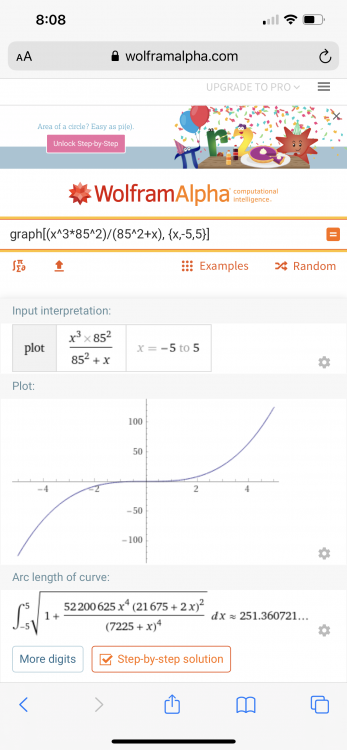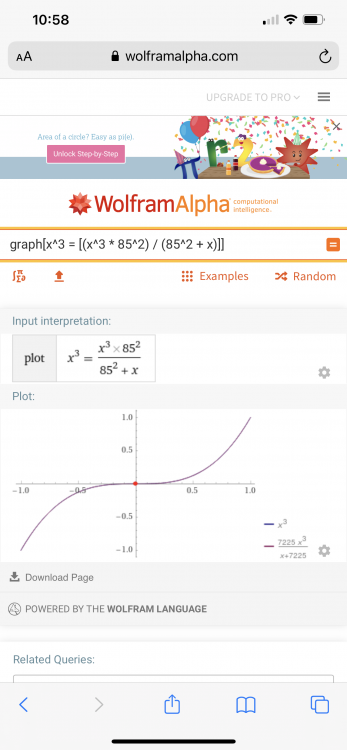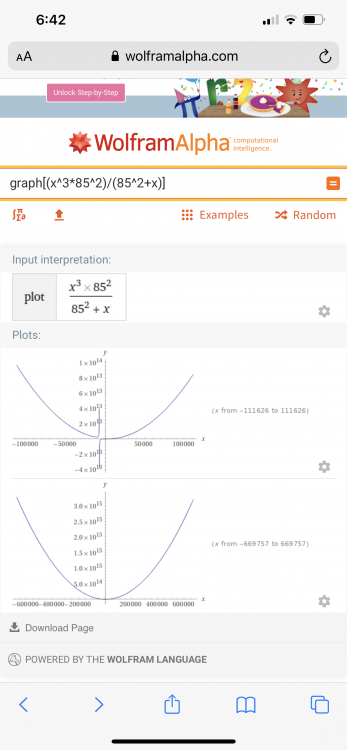##### Share on other sites

Posted (edited)
On 7/21/2021 at 3:10 AM, Trurl said:

There are 2 patterns. The one found in this graph where all possible x’s are graphed against a know pnp. And the other graphs that compare the known pnp to a calculated pnp.

This graph is the first case. It is definitely logarithmic. In fact, I think it is related to the normal distribution.

I show -5 to 5. Yes I know I must know x = 5 when looking at the graph. But you must admit when you plug and chug different values x, the results should be approaching x to the third.

The scales in the plots are very different, maybe you mean "patterns" are something like these approximations*? $\frac {x^3 * p^{2}}{ p^{2} + x} \approx \begin{cases}x^3 & x \ll p,p\gg0 \\x^2*p^2 & x \gg p^2,p \neq 0 \\0 & x \approx 0\end{cases}$.

Note the slightly more general expression using an arbitrary number p instead of 85.

I do not see a connection to primes.

*) I am not interested enough to try to provide a mathematical proof.

Edited by Ghideon
##### Share on other sites

No you are right there is no pattern in Primes in this graph. Instead it shows the value of the smaller semi-Prime. Pnp =x*y.

x^3 approximate -x^3

We subtract to make x appear around y equals zero so we know where to look for it.

The pattern of Primes comes from graphing all pnp’s.

If you know a number is a factor of a semi-Prime you know it is Prime.

I did not show that in the last graph. The last graph is to factor the semi-Prime.

##### Share on other sites

Ok you want a pattern. I saw a post here about a debate if math was created or invented. The same applies to patterns. The subconscious defines the pattern. I say a pattern is a pattern. I look for them. And share them with the goal that others will see them too.

But the fact is you should see the same pattern. Maybe someone has seen the pattern and is keeping it secret. In the hands of a capable mathematician this could be an attack on RSA.

But take the previous equation x^3 approx - x^3

And plug in a known x, say my favorite 5.

Let pnp = the y value of the graph

The hypothesis is that x of the graph will approach an x of zero where pnp of y equals a semiPrime.

In other words, when x =0 you will find every SemiPrime that has a factor of 5 will be found.

(You can invert x and y for y =0 so that the graph is y=f(x).)

##### Share on other sites

• 3 weeks later...

Can anyone fix this code? Shout out to Sensei.

2 lines of code in Mathematica. Variables can be floats. No errors just runs.

Clear x
Clear pnp

pnp = 85

For [x = 3 , x < (pnp/2), (x + 2);

[x] := If [{((x ^ 3  * pnp^2) / (pnp^2 + x) - x^3) < 1.0}]

]

##### Share on other sites

Thanks. The code is that simple. Put it seem to Print f of x instead of x.

In:= Clear x
Clear pnp

pnp = 85

f[x] = ((x ^ 3  * pnp^2) / (pnp^2 + x) - x^3)

x = 3; While [f[x_] < 1, Print[x]; (x + 2)]

Out= 3 Clear

Out= 85 Clear

Out= 85

Out= -(81/7228)

##### Share on other sites

On 8/11/2021 at 12:17 AM, Trurl said:

For [x = 3 , x < (pnp/2), (x + 2);

I think it should have comma at the end of the sentence, not semicolon.

   [x] := If [{((x ^ 3  * pnp^2) / (pnp^2 + x) - x^3) < 1.0}]

This line of code does not follow If[] statement format. It should be If [ condition, true, false ]

Your code has no two commas.

##### Share on other sites

• 3 weeks later...
Clear [x, pnp, f];

pnp = 85

For [x = 3, (x < (pnp/2)), (x = x + 2) ;
f[x] = x^4/(pnp^2 + x)
If[f[x] < 1,
Print[x]]
]

85

$RecursionLimit::reclim2: Recursion depth of 1024 exceeded during evaluation of If[f<1,Print[x]].$RecursionLimit::reclim2: Recursion depth of 1024 exceeded during evaluation of If[f<1,Print[x]].

$RecursionLimit::reclim2: Recursion depth of 1024 exceeded during evaluation of If[f<1,Print[x]]. General::stop: Further output of$RecursionLimit::reclim2 will be suppressed during this calculation.

Clear [x, pnp, y];

pnp = 85

For [x = 3, (x < (pnp/2)), (x = x + 2) ;
y = x^4/(pnp^2 + x)
Print[y]]

85

y

(125 Null)/1446

(2401 Null)/7232

(6561 Null)/7234

(14641 Null)/7236

(28561 Null)/7238

(10125 Null)/1448

(4913 Null)/426

(130321 Null)/7244

(194481 Null)/7246

(279841 Null)/7248

(3125 Null)/58

(531441 Null)/7252

(707281 Null)/7254

(923521 Null)/7256

(1185921 Null)/7258

(300125 Null)/1452

(1874161 Null)/7262

(2313441 Null)/7264

(2825761 Null)/7266


Well I think the syntax is correct now. Mathematica does not want to do what I tell it. I showed the Null because if you divide those values without the Null you get the answer.

I am only looking for x values that f[x] <1 (near zero). Again if anyone can tell me how to perform math on hundred-digit numbers let me know. Mathematica would handle it, but the recursion error is in my way. I'm so close. I want to be able to just cut in past pnp and find the zero values.

Also note the equation is simplified. That is thanks to an unanimous post. Mathematica simplified in one keyword what I was unable to do on paper.

I think I'm going to turn to free Linux opensource math programs.

Thanks Sensei for promptly answering my post. I think the problem is that Mathematica expects an integer value, but I have seen it use decimals many times before. It is just frustrating when the computer language doesn't do what you want. But I don't mind if someone programs it before I do, because that may lead to the Pappy Craylar Method being proven true.

##### Share on other sites

• 3 weeks later...
On 8/28/2021 at 1:59 AM, Trurl said:

I am only looking for x values that f[x] <1 (near zero). Again if anyone can tell me how to perform math on hundred-digit numbers let me know.

Please note what precision you need to get a useful outcome; truncation error and roundoff error may cause unwanted results. Not sure this will be an issue but the screenshots displays numbers that differs by a lot. Also note that there may be differences if you wish to perform integer or floating point arithmetics.

##### Share on other sites


PNP = 85

NSolve  [( x^4/(85^2 + x)) == 1]

85

{{x -> 9.22249}, {x -> -0.00294118 + 9.21954 I}, {x -> -0.00294118 -
9.21954 I}, {x -> -9.2166}}

Clear[x]
NSolve  [(
x^4/(35794234179725868774991807832568455403003778024228226193532908\
190484670252364677411513516111204504060317568667^2 + x)) == 1]

Clear[x]
NSolve  [(
x^4/(35794234179725868774991807832568455403003778024228226193532908\
190484670252364677411513516111204504060317568667^2 + x)) == 1]

Clear[x]
NSolve  [( x^4/((6863*7759)^2 + x)) == 1]

{{x -> 7297.26}, {x -> 0. + 7297.26 I}, {x ->
0. - 7297.26 I}, {x -> -7297.26}}

Clear[x]
NSolve  [( x^4/((6863*7759)^2 + x)) == 0.8]

{{x -> 6901.32}, {x -> 0. + 6901.32 I}, {x ->
0. - 6901.32 I}, {x -> -6901.32}}

I don't know why but Mathematica won't solve my large RSA number. Above are 3 different attempts. PNP=85 at 1, you have a value of 9.22249. This means the unknown x is less that 9.22249. Ignore the imaginary numbers because you should be only concerned with the real numbers. You can be sure that those weird values (often imaginary) are not the Prime factors.

Thanks for the reply, Ghideon. I think it is clear it works with small values. But as PNP gets larger, the error does not increase. The errors are the same. The error differs as the distance from x to PNP changes.

I don't yet know the error or the precision of float points. Mathematica should be able to crunch it. Look at the 3rd program paragraph. I tested an error of 0.8 from 0 to the computed value of the equation. I have never programmed hundred digit+ numbers. I think Mathematica will handle the calculation but as I show in the 2 program paragraph above it just refuses to compute. I thought that maybe someone on this forum would know how to program a hundred digit number and not have a error in floats. I know it is possible, but to use floats without rounding requires a program. I have looked for such programs in C++ but it was so complex. I chose Mathematica to have a ready made program, but it must not see the 100 digit number as a number.

But the problem is logic and not number crunching. If I am correct and the equation:  x^4/(85^2+x) error is always below 1 then we could pinpoint x.

##### Share on other sites

1 hour ago, Trurl said:
NSolve  [( x^4/(85^2 + x)) == 1]

NSolve[( x^4/(85^2 + x)) == 1,x]

Edited by Sensei
##### Share on other sites

22 hours ago, Trurl said:
NSolve  [(
x^4/(35794234179725868774991807832568455403003778024228226193532908\
190484670252364677411513516111204504060317568667^2 + x)) == 1]

Using @Sensei recommendation:

NSolve  [(x^4/(35794234179725868774991807832568455403003778024228226193532908190484670252364677411513516111204504060317568667^2 + x)) == 1,x]

Gives the result: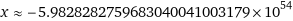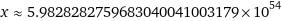(Note: I do not have access to a local installation, I used wolframalpha.com)

##### Share on other sites

On 9/15/2021 at 6:27 PM, Trurl said:

I am only looking for x values that f[x] <1 (near zero).

You can print a graph by typing the equation into Wolfram Alpha, and you will see (at least graphically) if it (or any equation) is near zero (or in any other range):

for equation "( x^4/(85^2 + x))"

## Create an account

Register a new account